• Call Now

1800-102-2727•

# Hybridization-Definition, Salient features, Types, Methods for finding hybridization and Hybridization involving d Orbitals

In day to day life, we are witnessed with a variety of colours around us. Don’t you find this interesting? Have you even wonder how we are able to see so many colours? This all happens because of hybridisation of universal colours to get variety of colours which makes our surrounding look more beautiful and colourful.

Let’s understand this with an example:
Start with one beaker of 50 mL red water that represents the 2s orbital and three beakers, each with 50 mL blue water, that represent three 2p orbitals. Mix all four beakers then we will get 200 mL of purple water.Why do we study hybridisation?

In methane, there should be one s-s overlapping and three -p  overlapping, for formation of four C - H bonds. it is assumed that the energy, and size of these orbitals should not be same but experimentally we found that all the bond  parameters of C - H bond in CH4 are same. why is this so? To figure this out, scientists had introduced a hypothetical concept which is known as hybridisation.

Table of content:

## What is hybridisation?

Hybridisation is defined as the concept of intermixing of atomic orbitals of similar or comparable energies of an atom to form a new set of almost same hybrid orbitals. The atomic orbitals of a similar energy level can only take part in hybridization and both fully filled and half-filled orbitals can also take part in this hypothetical process, provided they have comparable energy.
Example: Formation of SP2 hybridisationHybridisation = SP2

Example: Possible mixing of orbitals

##Salient features of hybridization

• Hybrid orbitals have the same shape and energy.
• Bonds formed by the hybrid orbitals are always more stable than bonds formed by the pure atomic orbital.
• The type of hybridization indicates the geometry of molecules.
• The number of hybrid orbitals is always equal to the number of atomic orbitals undergoing mixing. Also, hybrid orbitals never form pi bonds.

Note: Orientation of hybridized orbitals should be in a way to ensure minimum repulsion between electron pairs to obtain a stable geometry.

## Types of Hybridisation

Naming of Hybrid Orbitals: Naming can be done on the basis of the participating atomic orbitals in hybridisation.Based on the types of orbitals involved in mixing, hybridization can be classified as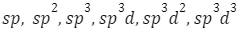• SP hybridisation : The intermixing of one S and one P-orbital of the comparable energy of the same shell of an atom to form two new equivalent orbitals is known as sp hybridisation. The new orbitals formed are known as sp hybridized orbitals and these forms linear geometry with an angle of 180°.• sp2 hybridisation: The intermixing of one s and two p-orbitals of comparable energy of the same shell of an atom to form three equivalent orbitals is known as sphybridisation. The new orbitals formed are known as sp2 hybrid orbitals. The geometry formed by this sp orbitals is trigonal planar and is maintained at 120°.• sp3 hybridisation: The intermixing of one s-orbital and three p-orbitals of comparable energy  belonging to the the same shell of an atom together to form four new equivalent orbitals is known as sp3 hybridization. The new orbitals formed are known as sp3 hybrid orbitals having geometry as tetrahedral.## Methods for finding hybridisation• Orbital box diagram method: An orbital diagram, also known as an orbital box diagram, is a representation of an atom's electronic arrangement. The electrons that fill the orbitals are shown by arrows (or half arrows).

Example of sp hybridization of BeCl2: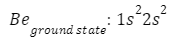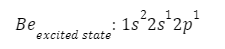Depicting the hybridization for different steric number

• Steric Number Method
What is the steric number?
Steric number is the number of atoms or group of atoms directly bonded to the central atom of a molecule plus the number of lone pairs attached to the central atom.• Example: Steric number of Methane, CH4
Steric number = 0 + 4
= 4

Hence, hybridisation of CH4 is SP3.

• Formula for steric number
To calculate the steric number, a mathematical expression used is: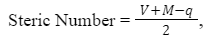where V = Number of valence electrons in the central atom
M = Number of surrounding monovalent atoms (H or halogens)
q = Charge on the ion
Example: Hybridisation of CO32-
Here central atom is.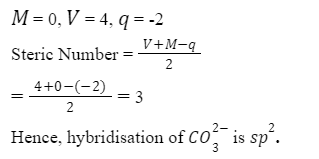## Hybridisation involving d-orbitals

In addition toand P orbitals, the elements in the third period have d orbitals. The energy of the 3d orbitals is similar to that of the 3s and 3p orbitals. 3d orbitals have an energy that is comparable to that of 4s and 4p orbitals. As a result, hybridization including 3s, 3p, and 3d, as well as 3d, 4s, and 4p, is possible.

•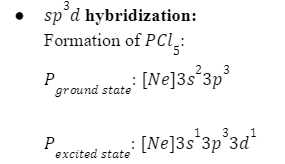•Hybridisation of Pcl5 is SP3d.• SP3d2 hybridization:
Formation of SF6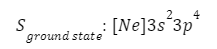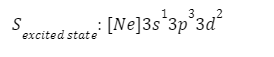Hybridisation of SF6 is SP3d2.

## Practice problems

Q1. Calculate thehybridisation of C02 using the concept of steric number.
Answer: Steric number = Number of sigma bond pairs + Number of lone pairsSteric number = 2 + 0 = 2  (since number of sigma bond is 2 and lone pair on C atom is 0)
For steric number equal to 2, the hybridisation comes out to be SP . Carbon dioxide (CO2) is therefore linear in geometry. The shape of CO2 is linear because there are no lone pairs affecting the orientation of the molecule.

Q2. The carbon atoms in acetylene are
A. SP
B. SP2
C. SP3
D. SP3d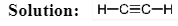The carbon atoms present in acetylene show sp hybridisation.
Using steric number, it can be easily calculated.
Steric number = Number of sigma bond pairs + Number of lone pairs

= 2 + 0
= 2
Hence, acetylene shows sp hybridisation.

Q3. The geometry of PlCO2 is
A. Tetrahedral
B. Trigonal planer
C. Square planer
D. Linear

By using steric number calculation we can predict the hybridisation and then the geometry of PlCO2.
Steric number = Number of sigma bond pairs + Number of lone pairs

Steric number = 3 sigma bond pairs + 0 lone pairs (Having 2 pi bonds)
= 3
The hybridisation comes out to be SP2
The geometry of PlCO2 is trigonal planar. Two oxygen atoms and one chlorine atom are placed at

the corners of an equilateral triangle.

Q4. What is the hybridisation of XeF4?
A. SP
B. SP2
C. SP3
D. SP3d2

Solution:  Using this formula of Steric Number =, we can calculate the hybridisation of XeF4
Number of valence electrons on the Xe atom (V) = 8
Number of surrounding monovalent atoms (M) = 4
There is no charge, q = 0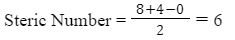Steric Number = 6 means the hybridisation is SP3d2.

Q1. What do you mean by orbital overlapping?

Answer: When two atoms are so close together, their atomic orbitals partially overlap. This partial merging of atomic orbitals is called orbital overlapping.

Q2. What are the different d-orbitals in d subshell?

Answer:  There are 5 d-orbitals in d subshell. These are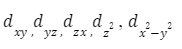Q3. What is the difference between geometry and shape?

Answer: Geometry of a molecule is the arrangement of orbitals containing lone pair and bond pair electrons around a central atom. Shape or structure is the arrangement of atoms around the central atom excluding the lone pairs.

Q4. Why does ammonia have a distorted tetrahedral geometry in spite of having sp3 hybridisation?

Answer: Ammonia has a distorted tetrahedral geometry and trigonal pyramidal shape. This is due to the presence of the lone pair that exerts greater lone pair-bond pair repulsion on the bonding orbitals. The bond angle in ammonia is 107°, which is less than the standard 109.5° for a tetrahedral molecule having sp3.

## Related topics:

 MOT VSEPR Theory Bond parameters VBT Metallic bonding Coordinate bondingTalk to our expert
Resend OTP Timer =
By submitting up, I agree to receive all the Whatsapp communication on my registered number and Aakash terms and conditions and privacy policy